Question

# A train is moving parallel and adjacent to a highway with a constant speed of 32 m/s.

A train is moving parallel and adjacent to a highway with a constant speed of 32 m/s. A car is traveling in the same direction as the train at 64 m/s. The car's horn sounds at 520 Hz and the train's whistle sounds at 320 Hz.

When the car is behind the train what frequency does an occupant of the car observe for the train whistle? The speed of sound is 343 m/s.

When the car is in front of the train what frequency does a train passenger observe for the car's horn?

The Doppler effect for the moving train is,

The frequency of the observer f11=520HZ

The frequency of the source is f1=320HZ

velocity of the source is v+=32m/s

velocity of the observer is v-=64m/s

Then, f11=f1(v+/-v0)/(v-/vs)

520=320(32/-v0)/(64/vs)

-vs/v0=3.25m/s

But we want to the observer frequency of the car observing train whistle frequency is,

f11=f1(v+/-vo)/(v-/+vs)

=320(-32/343)(3.25)

=97HZ

so the frequency of the train whistle observed by the car is 97HZ

#### Earn Coins

Coins can be redeemed for fabulous gifts.

Similar Homework Help Questions
• ### A train is moving past a crossing where cars are waiting for it to pass. While...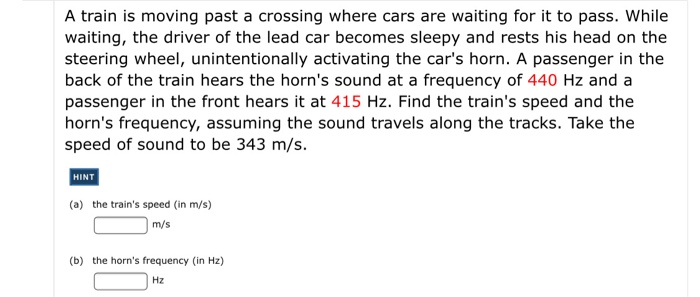A train is moving past a crossing where cars are waiting for it to pass. While waiting, the driver of the lead car becomes sleepy and rests his head on the steering wheel, unintentionally activating the car's horn. A passenger in the back of the train hears the horn's sound at a frequency of 440 Hz and a passenger in the front hears it at 415 Hz. Find the train's speed and the horn's frequency, assuming the sound travels along...

• ### A train is moving pass a crossing where cars are waiting for it to pass. While...

A train is moving pass a crossing where cars are waiting for it to pass. While waiting, the driver of the lead car becomes sleepy and rests his head on the steering wheel, unintentionally activating the car's horn. A passenger in the back of the train hears the horn's sound at a frequency of 444 Hz and a passenger in the front hears it at 415 Hz. Find the train's speed and the horn's frequency, assuming the sound travels along...

• ### Two trains approach each other on separate but adjacent tracks. Train 1 is traveling at a...

Two trains approach each other on separate but adjacent tracks. Train 1 is traveling at a speed of 35.9 m/s and train 2 at a speed of 27.6 m/s. If the engineer of train 1 sounds his horn which has a frequency of 520 Hz, determine the frequency of the sound heard by the engineer of train 2. (Use 343 m/s as the speed of sound. Enter your answer to the nearest Hz.)

• ### A car is parked 20.0 m directly south of a railroad crossing. A train is approaching...

A car is parked 20.0 m directly south of a railroad crossing. A train is approaching the crossing from the west, headed directly east at a speed of 55.5 m/s. The train sounds a short blast of its 312-Hz horn when it reaches a point 20.0 m west of the crossing. What frequency does the car's driver hear when the horn blast reaches the car? The speed of sound in air is 343 m/s. (Hint: Assume that only the component...

• ### A train: A enters a train station with constant speed of .00 m/s while another train...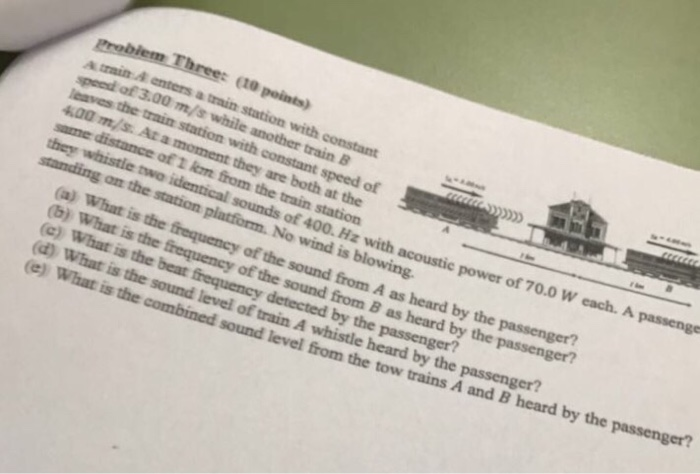A train: A enters a train station with constant speed of .00 m/s while another train B leaves the train station with constant speed of 4.00 m/s. At a moment they are both at the same distance of 1 km from the train station they whistle two identical sounds of 400. Hz with acoustic power of 70.0 W each. A passenger standing on the station platform. No wind is blowing. (a) What is the frequency of the sound from A...

• ### A car drives at a speed of 25 m/s along a road parallel to a railroad...

A car drives at a speed of 25 m/s along a road parallel to a railroad track. A train traveling at 15 m/s sounds a horn that vibrates at 300 Hz. (a) If the train and car are moving toward each other, what frequency of sound is heard by a person in the car? (b) If the train and car are moving away from each other, what frequency of sound is heard in the car?

• ### At rest, a car's horn sounds the note A (440 Hz). The horn is sounded while...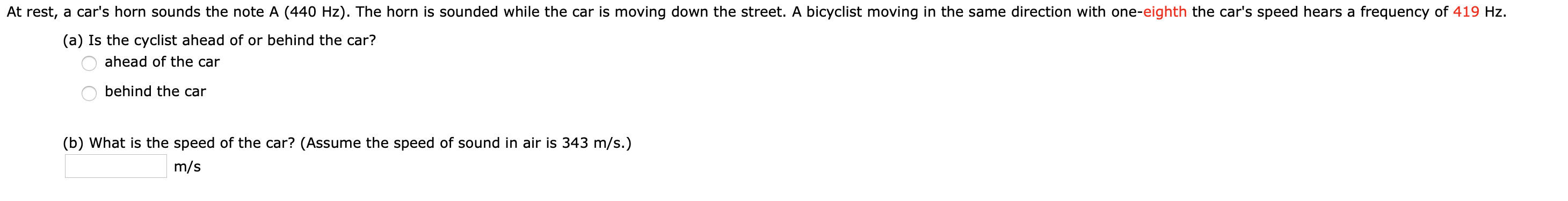At rest, a car's horn sounds the note A (440 Hz). The horn is sounded while the car is moving down the street. A bicyclist moving in the same direction with one-eighth the car's speed hears a frequency of 419 Hz (a) Is the cyclist ahead of or behind the car? ahead of the car behind the car (b) What is the speed of the car? (Assume the speed of sound in air is 343 m/s.) m/s

• ### At rest, a car's horn sounds the note A (440 Hz). The horn is sounded while...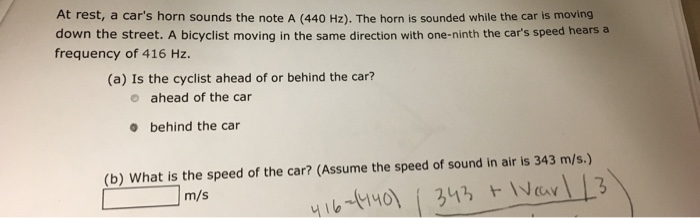At rest, a car's horn sounds the note A (440 Hz). The horn is sounded while the car is moving down the street. A bicyclist moving in the same direction with one-ninth the car's speed hears a frequency of 416 Hz. (a) Is the cyclist ahead of or behind the car? e ahead of the car behind the car behind the car What is the speed of the c, r? (Assume the speed of sound in air is 343 m/s.)...

• ### At rest, a car's horn sounds the note A (440 Hz). The horn is sounded while...At rest, a car's horn sounds the note A (440 Hz). The horn is sounded while the car is moving down the street. A bicyclist moving in the same direction with one-eighth the car's speed hears a frequency of 419 Hz. (a) Is the cyclist ahead of or behind the car? o ahead of the car O behind the car (b) What is the speed of the car? (Assume the speed of sound in air is 343 m/s.) m/s

• ### A train is approaching a signal tower at a speed of 40 m/s. The train engineer...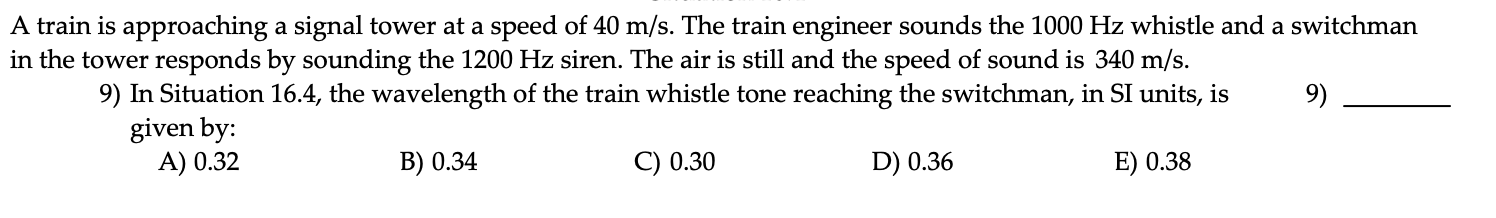A train is approaching a signal tower at a speed of 40 m/s. The train engineer sounds the 1000 Hz whistle and a switchman in the tower responds by sounding the 1200 Hz siren. The air is still and the speed of sound is 340 m/s. 9) In Situation 16.4, the wavelength of the train whistle tone reaching the switchman, in SI units, is given by: A) 0.32 B) 0.34 C) 0.30 D) 0.36 E) 0.38 A train is approaching...